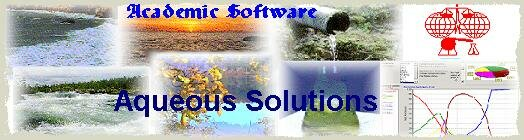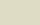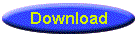Software for the quantitative treatment of equilibria in solution

Aq_Solutions is a suite of programs for all scientists interested in the quantitative treatment of equilibria in solution. These will be important tools for all scientists working in environmental, medical, industrial and educational fields. They have been developed with help and encouragement from the Analytical Division of IUPAC.

The suite consists of a controlling program (Aq_Solutions.exe). This gives access to a suite of programs which, it is planned, will be regularly extended and up-dated. The principle authors are Igor V. Sukhno,Vladimir Y. Buzko (Krasnodar, Russia) and Leslie D.Pettit, Leeds, UK.

Programs include:

 SIT To correct stability constants for changes in ionic strength using SIT (Specific Interaction Theory) and to estimate SIT parameters with full statistics. It contains an editable database of published SIT parameters. It also provides routines to inter-convert MolaRities (c) and MolaLities (m), and lg K(c) and lg K(m). A version of the SIT program to correct stability constants for changes in seawater salinity and in electrolyte mixtures (fluids) is in preparation. Electrolytes To calculate electrolyte activity coefficients, osmotic coefficients and the activity of water using Pitzer and the Lin-Tseng-Lee equations. Also to calculate ionic activity coefficients using the Millero-Pitzer method. A version of the Electrolytes program to calculate the ionic activity coefficients for the most important ions in seawater is in preparation. Acid-base Acid-base: To calculate the composition of acid-base systems at equilibrium in electrolyte solutions and seawater. To also calculate H+ activity coefficients for correction of paH to p[H+] in different ionic media. The concept of apparent equilbrium constant is used to calculate equilibrium constants for more than 260 mono and polycarboxylic acids with background ions. A version of the Acid-base program to calculate the H+ activity coefficient in seawater at different salinity (chlorinity) and at different temperature is in preparation. Temperature effects Temperature effects: To study and predict the temperature dependence of lg K using eight thermodynamic equations: Van't Hoff equation Isobar equation Extended Isobar equation Clarke-Glew equation Extended Clarke-Grew equation DQUANT equation Valentiner equation Blandamer-Robertson-Scott equation Full statistics are included. Gas solubility To calculate O2 solubility in water, 22 electrolyte solutions, natural fluids and seawater as a function of temperature, concentration and salinity. A program to calculate the solubilities of other gasses is in preparation. Speciation To calculate & display species distribution curves for complexes (including insoluble species). Speciation may be displayed as a function of pH, pL (any reactant) or total reactant concentration and as either percentages or as species concentrations (log values). When insoluble phases are present lg S values may also be plotted. Both graphs and tables of numeric values may be displayed, printed or saved to disk. The sensitivity of speciation curves to errors in lg K values may be demonstrated graphically. Titration simulations To simulate M-L titration curves in real time and display speciation dynamically. Species L, HL, H2L, ML, ML2, MHL and MH-1L may be included and stability constants adjusted in real time. Titration curves, speciation curves and pi-charts are calculated in real time.

In addition, other programs are in preparation. and will be included at a later date.
These include programs for electrode calibration, for properties of individual electrolyte solutions and their mixtures (solubility, density, viscosity, DCp, freezing-point, boiling-point, surface tension), for water properties as a function of temperature, pressure and concentration of individual electrolytes or electrolyte mixtures (aw, pKw, DHw, DCp, viscosity, density).

Download Aq_solutions as a .zip file (7 MB). Unpack, retaining the directory structure.